# Chapter 8.2. Preparation for a Practical Exam – Part II

In this chapter, we will look at a practical exam held at SoftUni on December 18, 2016. The problems give a good idea of what we can expect at the practical exam in programming at SoftUni.

## Exam Problems

Traditionally, the Practical Exam of SoftUni consists of 6 practical programming problems:

• Simple problem (without checks).
• A problem with a single condition.
• A problem with more complex conditions.
• A problem with a single loop.
• A problem with nested loops (drawing a figure on the console).
• A problem with nested loops and more complex logic.

Let's look at a real exam topic, the problems it contains, and their solutions.

### Problem: Distance

Write a program that calculates what is the distance passed by a car (in kilometers), for which we know the initial speed (km/h), the initial time frame in minutes, then the speed is increased by 10%, the second time frame, then the speed is decreased by 5%, and the time until the end of the trip. To calculate the distance, you need to convert the minutes into hours (e.g., 70 minutes = 1.1666 hours).

### Input Data

The input is read from the console and consists of 4 lines:

• The initial speed in km/h – an integer within the range [1 … 300].
• The first time in minutes – an integer within the range [1 … 1000].
• The second time in minutes – an integer within the range [1 … 1000].
• The third time in minutes – an integer within the range [1 … 1000].

### Output Data

Print a number on the console: kilometers passed, formatted up to the second character after the decimal point.

### Sample Input and Output

90
60
70
80
330.90 Distance with initial speed: 90 km/h * 1 hour (60 min) = 90 km
After speed increase: 90 + 10% = 99.00 km/h * 1.166 hours (70 min) = 115.50 km
After speed decrease: 99 - 5% = 94.05 km/h * 1.33 hours (80 min) = 125.40 km
Total number of km passed: 330.9 km
140
112
75
190
917.12 Distance with initial speed: 140 km/h * 1.86 hours (112 min) = 261.33 km
After speed increase: 140 + 10% = 154.00 km/h * 1.25 hours (75 min) = 192.5 km
After speed decrease: 154.00 - 5% = 146.29 km/h * 3.16 hours (190 min) = 463.28 km
Total number of km passed: 917.1166 km

## Hints and Guidelines

Such a description may look misleading and incomplete at first glance, which adds to the complexity of a relatively easy problem. Let's separate the problem into a few sub-problems and try to solve each problem one by one, which will lead us to the final result:

• Our initial sub-problem will be to read the input data entered by the user and store them in appropriate variables.
• Execution of the main programming logic, which in our case is a batch of simple calculations of the data that we already have.
• Caculate and format the result.

The main part of the programming logic is to calculate what will be the distance passed after all changes in speed. As during execution of the program, part of the data that we have is modified, we could separate the program code into a few logically separated parts:

• Calculation of the distance passed with initial speed.
• Change of speed and calculation of the distance passed.
• Last change of speed and calculation.

For reading data from the console, we use Scanner: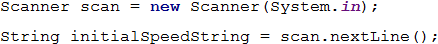By the problem condition, input data is being entered on four separate rows, therefore, we should execute the previous code a total of four times.To perform calculations, we choose to use a type double.

This way, we successfully solved the first sub-problem. The next step is to convert the input data into appropriate types to perform the needed calculations. We choose to use Integer or int type because the condition of the problem says that the input data must be within a particular range, for which this data type is sufficient. We will do the conversion in the following way: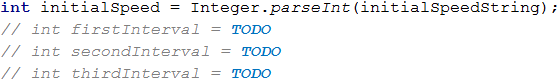Initially, we save a variable that we will use multiple times. This approach of centralization gives us flexibility and the opportunity to change the overall result of the program with minimal effort. In case, we need to change the value, we need to do it only in one place in the code, which saves us time and effort.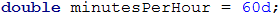Avoiding repetitive code (centralization of program logic) in the problems that we examine in the present book may look unnecessary at first glance, but this approach is very important upon building large-scale applications in a real work environment, and practicing it in an initial stage of training will help you build a quality programming style.

We calculate the travel time (in hours) by dividing the time by 60 (minutes in an hour). The travel distance is calculated by multiplying the starting speed by the time passed (in hours). After that, we change the speed by increasing it by 10%, as per the problem description. Calculating the percentage, as well as the following distances passed, is done in the following way:

• The time interval (in hours) is calculated by dividing the provided time interval in minutes by the minutes in an hour (60).
• The distance passed is calculated by multiplying the time interval (in hours) by the speed obtained after the increase.
• The next step is to decrease the speed by 5%, as per the problem description.
• We calculate the remaining distance as described in the first two points.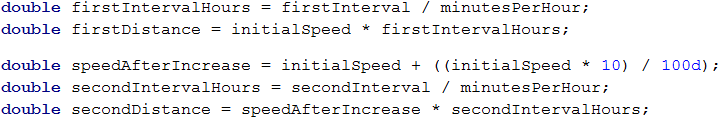So far, we solved two of the most important sub-problems, namely the data input and their processing. What remains is to calculate the result. Since it is required to format it up to 2 characters after the decimal point, we can do it as follows: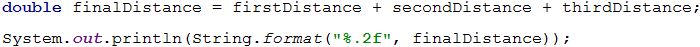If you have worked correctly and run the program with the input data from the problem condition, you will see that it works correctly.

### Problem: Changing Tiles

Haralambi has some savings that he wants to use to change the tiles on the bathroom floor. The floor is rectangular, and the tiles are triangular. Write a program that calculates if his savings will be sufficient. Read from the console the width and length of the floor, as well as one of the sides of the triangle with its height to it. We must calculate how many tiles are needed, to cover the floor. The number of tiles must be rounded up to the higher integer and 5 more tiles must be added as spare tiles. Also, read from the consolethe price per tile and the amount paid for the work** of a workman.

### Input Data

The following 7 lines are read from the console:

• The savings
• The width of the floor
• The length of the floor
• The side of the triangle
• The height of the triangle
• The price of a tile
• The amount for the workman

All numbers are real numbers in the range [0.00 … 5000.00].

### Output Data

The following must be printed on the console as a single line:

• If the money is sufficient:
• “{The remaining money} lv left.”
• If the money IS NOT sufficient:
• “You'll need {Needed money} lv more.”

The result must be formatted up to the second character after the decimal point.

### Sample Input and Output

500
3
2.5
0.5
0.7
7.80
100
25.60 lv left. Floor area → 3 * 2.5 = 7.5
Tile area → 0.5 * 0.7 / 2 = 0.175
Needed tiles → 7.5 / 0.175 = 42.857… = 43 + 5 spare tiles = 48
Total amount → 48 * 7.8 + 100 (workman) = 474.4
474.4 < 50025.60 lv left
1000
5.55
8.95
0.90
0.85
13.99
321
You'll need 1209.65 lv more. Floor area → 5.55 * 8.95 = 49.67249
Tile area → 0.9 * 0.85 / 2 = 0.3825
Needed tiles → 49.67249 / 0.3825 = 129.86… = 130 + 5 spare tiles = 135
Total amount → 135 * 13.99 + 321 (workman) = 2209.65
2209.65 > 10001209.65 lv are insufficient

## Hints and Guidelines

The problem requires our program to accept more input data and perform more calculations, even though the solution is identical. Reading the input data is done familiarly. Note that the Input part of the condition states that all input data are real numbers, and for that reason, we would use the decimal type.

Now that we already have everything to execute the programming logic, we can move to the next part. How can we calculate what is the needed number of tiles that will be sufficient to cover the entire floor? The condition is that tiles have a triangular shape, which can confuse, but practically, the problem needs just basic calculations. We can calculate the common part of the floor by the formula for finding rectangle area, and the area of a single tile using the relevant formula for triangle area.

To calculate the number of tiles that are needed, we divide the floor area by the area of a single tile (we should not forget to add the 5 additional tiles, that were mentioned in the condition).Pay attention that the condition state that we should round up the number of tiles, obtained upon the division, up to the higher number, and then we should add 5. Find more information about the system functionality that does that: Math.ceil(…).

We can find the result by calculating the total amount needed to cover the entire floor, by adding the tile price with the price for the workman, which we have from the input data. We can guess that the total costs for tiles can be calculated by multiplying the number of tiles by the price per tile. Whether the amount that we have will be sufficient, we understand by comparing the savings (from the input data) and the total costs.

### Problem: Flowers

A flowers shop offers 3 types of flowers: chrysanthemums, roses, and tulips. The prices depend on the season.

Season Chrysanthemums Roses Tulips
spring / summer
autumn / winter
2.00 lv/pc
3.75 lv/pc
4.10 lv/pc
4.50 lv/pc
2.50 lv/pc
4.15 lv/pc

On holidays, the prices of all flowers are increased by 15%. The following discounts are offered:

• For purchasing more than 7 tulips in spring – 5% of the price of the whole bouquet.
• For purchasing 10 or more roses in winter – 10% of the price of the whole bouquet.
• For purchasing more than 20 flowers in total in any season – 20% of the price of the whole bouquet.

Discounts are made in the above-described order and can be combined! All discounts are valid after the price increase for a holiday!

The price for arranging a bouquet is always 2 lv. Write a program that calculates the price of a bouquet.

### Input Data

The input is read from the console and contains exactly 5 lines:

• The first line contains the number of purchased chrysanthemums – an integer within the range [0 … 200].
• The second line contains the number of purchased roses – an integer within the range [0 … 200].
• The third line contains the number of purchased tulips – an integer within the range [0 … 200].
• The fourth line shows the season – [Spring, Summer, Autumn, Winter].
• The fifth line specifies if the day is a holiday – [Y = yes / N = no].

### Output Data

Print on the console 1 number – the price of flowers, formatted up to the second character after the decimal point.

#### Sample Input and Output

2
4
8
Spring
Y
46.14 Price: 2*2.00 + 4*4.10 + 8*2.50 = 40.40 lv
Holiday: 40.40 + 15% = 46.46 lv
5% discount for more than 7 tulips in spring: 44.14lv
The flowers are in total 20 or less: no discount
44.14 + 2 for arranging the bouquet = 46.14 lv
3
10
9
Winter
N
69.39 Price: 3*3.75 + 10*4.50 + 9*4.15 = 93.60 lv
Not a holiday: no increase in price
10% discount for 10 or more roses in winter: 84.24lv
Total flowers more than 20: 20% discount = 67.39lv
67.39 + 2 for arranging the bouquet = 69.39 lv
Input Output
10
10
10
Autumn
N
101.20

### Hints and Guidelines

After carefully reading the condition, we understand that we need to do simple calculations, but this time we will need additional logical checks. We need to pay more attention to the moment of making changes in the final price so that we can properly build the logic of our program. Again, the bold text gives us sufficient guidelines on how to proceed. To begin with, we will separate the already defined values into variables, as we did in the previous problems: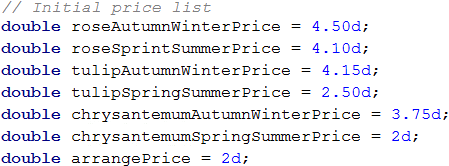We do the same for the rest of the defined values: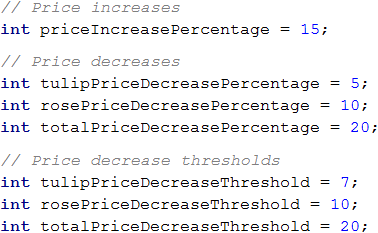Our next sub-problem is to read correctly the input data from the console. We will do it familiarly, but this time we will combine two separate functions – one for reading a line from the console and another one for its conversion into a numeric data type: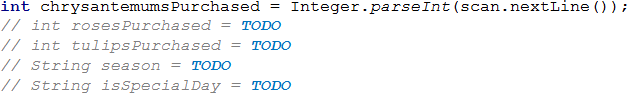Let's think of the most appropriate way to structure our programming logic. It is clear from the condition that the path of the program is divided mainly into two parts: spring / summer and autumn / winter. We can do the separation by conditional construction, by storing variables in advance for the prices of the individual flowers and the final result.What remains is to perform a few checks regarding the discounts of the different types of flowers, depending on the season, and to modify the final result.

### Testing in The Judge System

Write a program that calculates statistics for grades in an exam. In the beginning, the program reads the number of students who attended the exam and for each student – their grade. In the end, the program must print the percentage of students that have grades between 2.00 and 2.99, between 3.00 and 3.99, between 4.00 and 4.99, 5.00 or more, and the average grade of the exam.

### Input Data

Read from the console a sequence of numbers, each on a separate line:

• On the first line – the number of students who attended the exam – an integer within the range [1 … 1000].
• For each student on a separate line – the grade on the exam – a real number within the range [2.00 … 6.00].

### Output Data

Print on the console 5 lines that hold the following information:

• "Top students: {percentage of students with grade of 5.00 or more}%".
• "Between 4.00 and 4.99: {between 4.00 and 4.99 included}%".
• "Between 3.00 and 3.99: {between 3.00 and 3.99 included}%".
• "Fail: {less than 3.00}%".

The results must be formatted up to the second symbol after the decimal point.

### Sample Input and Output

10
3.00
2.99
5.68
3.01
4
4
6.00
4.50
2.44
5
Top students: 30.00%
Between 4.00 and 4.99: 30.00%
Between 3.00 and 3.99: 20.00%
Fail: 20.00%
Average: 4.06
5 or more: 3 students = 30% of 10
Between 4.00 and 4.99 - 3 students = 30% of 10
Between 3.00 and 3.99 - 2 students = 20% of 10
Under 3 - 2 students = 20% of 10
The average grade is: 3 + 2.99 + 5.68 + 3.01 + 4 + 4 + 6 + 4.50 + 2.44 + 5 = 40.62 / 10 = 4.06
Input Output
6
2
3
4
5
6
2.2
Top students: 33.33%
Between 4.00 and 4.99: 16.67%
Between 3.00 and 3.99: 16.67%
Fail: 33.33%
Average: 3.70

### Hints and Guidelines

From the condition, we see that we will read the number of students, and then, their grades. For that reason first, we will read the number of students and save it in a variable of int type. To read and process the grades themselves, we will use a for loop. The value of the int variable will be the end value of the i variable from the loop. This way, at each iteration of the loop, will read each one of the grades.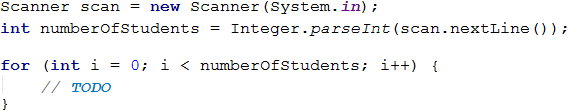Before executing the code of the for loop, we will create variables where we will store the number of students for each group: poor results (up to 2.99), results from 3 to 3.99, from 4 to 4.99, and grades above 5. We will also need one more variable, where we will store the sum of all grades, via which we will calculate the average grade of all students.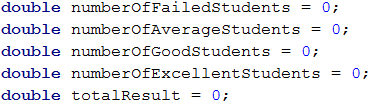We run the loop, and inside it, we declare one more variable, in which we will store the currently entered grade. The variable will be double type, and upon each iteration, we will check what is its value. According to this value, we increase the number of students in the relevant group by 1, as we should not forget to increase the total amount of the grades, which we also track.What percentage is occupied by a group of students from the total number, we can calculate by multiplying the number of students from the respective group by 100, and then dividing by the total number of students.Pay close attention to the numeric data type that you work with upon doing these calculations.

The final result is formed in the well know fashion – up to the second character after the decimal point.

### Problem: Christmas Hat

Write a program that reads from the console an integer n and draws a Christmas hat with a width of 4 * n + 1 columns and a height of 2 * n + 5 rows, as in the examples below.

### Input Data

The input is read from the console – an integer n within the range [3 … 100].

### Output Data

Print on the console a Christmas hat, exactly like in the examples.

### Sample Input and Output

Input Output
4 ......./|\.......
.......\|/.......
.......***.......
......*-*-*......
.....*--*--*.....
....*---*---*....
...*----*----*...
..*-----*-----*..
.*------*------*.
*-------*-------*
*****************
*.*.*.*.*.*.*.*.*
*****************
Input Output
7 ............./|\.............
.............\|/.............
.............***.............
............*-*-*............
...........*--*--*...........
..........*---*---*..........
.........*----*----*.........
........*-----*-----*........
.......*------*------*.......
......*-------*-------*......
.....*--------*--------*.....
....*---------*---------*....
...*----------*----------*...
..*-----------*-----------*..
.*------------*------------*.
*-------------*-------------*
*****************************
*.*.*.*.*.*.*.*.*.*.*.*.*.*.*
*****************************

### Hints and Guidelines

In problems requiring drawing on the console, most often the user inputs an integer that is related to the total size of the figure that we need to draw. As the problem requirements mention how the total length and width of the figure are calculated, we can use them as starting points. In the examples, it is clear that regardless of the input data, we always have the first two rows that are almost identical.

......./|\.......
.......\|/.......

We also notice that the last three rows are always present, as two of them are completely the same.

*****************
*.*.*.*.*.*.*.*.*
*****************

By these observations, we can come up with the formula for the height of the variable part of the Christmas hat. We use the formula specified in the problem to calculate the total height, by subtracting the size of the unchangeable part. We get (2 * n + 5) – 5 or 2 * n.

To draw the dynamic or the variable part of the figure, we will use a loop. The size of the loop will be from 0 to the width that we have by requirements, namely 4 * n + 1. Since we will use this formula in a few places in the code, it is a good practice to declare it in a separate variable. Before running the loop, we should declare variables for the number of individual symbols that participate in the dynamic part: dots and dashes. By analyzing examples, we can also prepare formulas for the starting values of these variables. Initially, the dashes are 0, but we can calculate the number of dots by subtracting 3 from the total width (the number of symbols that are building the top of the Christmas hat) and then dividing by 2, as the number of dots on both sides of the hat is the same.

.......***.......
......*-*-*......
.....*--*--*.....
....*---*---*....
...*----*----*...
..*-----*-----*..
.*------*------*.
*-------*-------*

All that remains is to execute the body of the loop, as after each drawing we decrease the number of dots by 1 and increase the number of dashes by 1. Let's not forget to draw one star between each of them. The sequence of drawing in the body of the loop is the following:

• Symbol string of dots
• Star
• Symbol string of dashes
• Star
• Symbol string of dashes
• Star
• Symbol string of dots

In case we have worked correctly, we will obtain figures identical to those in the examples.

### Problem: Letters Combinations

Write a program that prints on the console all combinations of 3 letters within a specified range by skipping the combinations containing a certain letter. Finally, print the number of printed combinations.

### Input Data

The input is read from the console and contains exactly 3 lines:

• A small letter from the English alphabet for a beginning of the range – between 'a' and 'z'.
• A small letter from the English alphabet for the end of the range – between the first letter and 'z'.
• A small letter from the English alphabet – from 'a' to 'z' – as the combinations containing this letter are skipped.

### Output Data

Print on a single line all combinations, corresponding to the requirements, followed by their number, separated by a space.

### Sample Input and Output

a
c
b
aaa aac aca acc caa cac cca ccc 8 All possible combinations with letters 'a', 'b' and 'c' are:
aaa aab aac aba abb abc aca acb acc baa bab bac bba bbb bbc bca bcb bcc caa cab cac cba cbb cbc cca ccb ccc
The combinations containing 'b' are not valid.
8 valid combinations remain.
Input Output
f
k
h
fff ffg ffi ffj ffk fgf fgg fgi fgj fgk fif fig fii fij fik fjf fjg fji fjj fjk fkf fkg fki fkj fkk gff gfg gfi gfj gfk ggf ggg ggi ggj ggk gif gig gii gij gik gjf gjg gji gjj gjk gkf gkg gki gkj gkk iff ifg ifi ifj ifk igf igg igi igj igk iif iig iii iij iik ijf ijg iji ijj ijk ikf ikg iki ikj ikk jff jfg jfi jfj jfk jgf jgg jgi jgj jgk jif jig jii jij jik jjf jjg jji jjj jjk jkf jkg jki jkj jkk kff kfg kfi kfj kfk kgf kgg kgi kgj kgk kif kig kii kij kik kjf kjg kji kjj kjk kkf kkg kki kkj kkk 125
Input Output
a
c
z
aaa aab aac aba abb abc aca acb acc baa bab bac bba bbb bbc bca bcb bcc caa cab cac cba cbb cbc cca ccb ccc 27

### Hints and Guidelines

According to the condition, we have as input data 3 lines, each of which is represented by one character of the ASCII table (https://www.asciitable.com). We could use an already defined function in Java to read a single symbol from the console and save it in a variable with type char: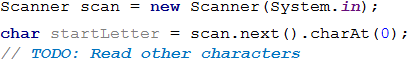Let's think of how we can achieve the final result. The problem condition is to print all characters from the starting to the end (by skipping a particular letter), what should we do?

The easiest and most efficient way is to use a loop, by iterating through all characters and printing those that are different from the letter that we need to skip. One of the advantages of Java is that we have the opportunity to use a different data type for a loop variable: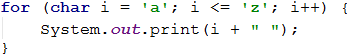The result of running the code is all letters from a to z included, printed on a single line and separated by spaces. Does this look like the final result of our problem? We must find a way to print 3 characters, as required, instead of 1. The execution of the program is very similar to a gaming machine. We usually win there if we manage to arrange several identical symbols. Let's say that the machine has space for three characters. When we stop on a particular character in the first place, the other two places will continue rolling characters among all possible ones. In our case, all possible characters are the letters from the starting to the end one, entered by the user, and the solution of our program is identical to the way a gaming machine works.

We use a loop that runs through all characters from the starting to the end letter, included. On each iteration of the first loop, we run a second one with the same parameters (but only if the letter of the first loop is valid, i.e., does not match the one that we must exclude, by requirements). In each iteration of the second loop, we run one more with the same parameters and the same condition. This way, we have three nested loops, as we will print the characters in the body of the latter.Let's not forget that we also need to print the total number of valid combinations that we have found, and they must be printed on the same line, separated by a space.